# MA2051 - Ordinary Differential EquationsSample ExamOrginally Given 1996 D Term

Instructions:  Do your work on the paper provided.  Put your
name and section number on the cover and on each page.  Work neatly
and show your work.
Remember, your work and explanations are graded, not just the
final answer.

1. (20 points) Solve each of the following initial value problems: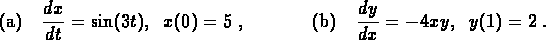2. (35 points) In this problem, you will analyze the following linear differential equation:(a)
Find a nontrivial solution for the corresponding homogeneous equation.
(b)
Find a particular solution for the original differential equation.
(c)
Find the solution for the differential equation which satisfies the initial condition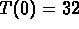.
(d)
Can you choose the initial condition so that the solution to the initial value problem contains only the particular solution? Explain your answer clearly.

3. (35 points) A large lecture hall containing 40,000 cubic meters of air is originally free of carbon monoxide. Beginning at time t=0, smoke containing 3% carbon monoxide begins leaking into the hall at the rate of 2 cubic meters per minute. The ventilation system removes the mixture of air and smoke from the hall at the same rate (2 cubic meters per minute).

(a)
Write an expression for the rate at which carbon monoxide is entering the room.
(b)
Write an expression for the rate at which carbon monoxide is leaving the room. (Your expression should depend on the concentration of carbon monoxide in the room at time t.) Why is it important to assume that the smoke and air are ``thoroughly mixed'' before the mixture is removed from the room?
(c)
Write a balance equation that relates the net change in the amount of carbon monoxide in the hall over a time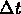with the rate at which it is added and lost.
(d)
Use the results from parts (a), (b) and (c) to derive a differential equation model for the concentration of carbon monoxide in the lecture hall at time t. (Do not solve the differential equation.)

4. (10 points) The differential equation in Problem 2 models heat-loss with a non-constant outside temperature (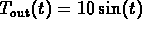). Make a rough sketch of the solution curve for this model with initial data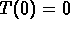. Does the inside temperature oscillate with the same range as the outside temperature (degrees)? (Hint: You don't need the solution formula. Sketch the outside temperature first and determine where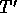is positive, negative, and zero.)

Explain why it is not possible for the solution curve to follow the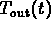exactly.

```

© 1997 by MA2051 Webmasters

All rights Reserved. File last modified on January 31, 1997

```